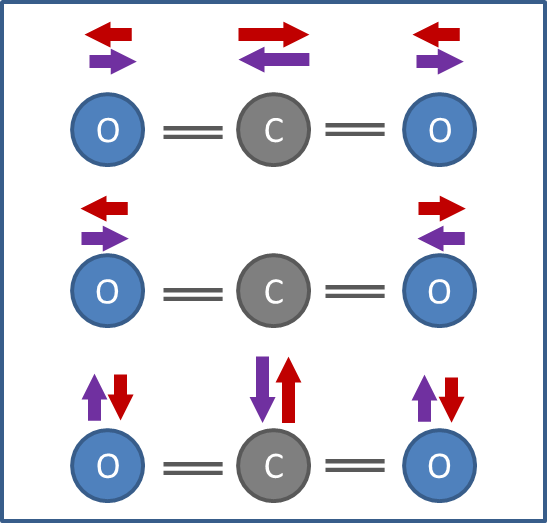0 Rates

Quantum physics at work: Infrared spectroscopy

We experience quantum physics in many everyday situations, usually without even knowing it. Without it there would be no internet, no computers, no magnetic resonance images of our bodies, no modern medicines. Infrared spectroscopy, a frequently applied method for chemical analysis, shows the peculiarities of quantum physics in a particularly impressive way.

How does infrared spectroscopy work? A sample is irradiated with a wide-band infrared light in the wavelength range of 2.5 to 25 µm. The light passes through the sample or, alternatively, is reflected from the sample. This light is then split into its spectral components. Information about the sample can be gained from this spectrum. To understand which information can be gained from this spectrum we will look at a simple and very common molecule: carbon dioxide (CO2).

Molecules are mechanically vibrating entities. They can vibrate in various ways, as shown in Figure 1 for the CO2 molecule. First we will look at the molecular vibration shown in the top line of Figure 1. This is called the asymmetric stretching vibration. The resonance frequency of this vibration is at 70.4 terahertz, the corresponding wavelength therefore being 4.26 µm. The positive center of charge of the molecule, which is generated by the charge of the atomic nuclei, and the negative center of charge, which comes from the electrons, move periodically towards and away from each other during this vibration. This creates a vibrating electrical dipole – a sort of “antenna” – which is why this vibration is infrared active. The molecule can take up an infrared photon of this wavelength from an electromagnetic radiation field and thus moves from the ground state to the excited state – or it can radiate off an infrared photon to move from the excited state back to the ground state. Due to the laws of quantum physics the vibration states are quantized, as will be shown in more detail later using the example of the rotational levels.Figure 1: The normal modes of vibration of a CO2 molecule. Top: Asymmetric stretching vibration. Middle: Symmetric stretching vibration. Bottom: Deformation vibration

Determining the concentration

The number of infrared photons of this wavelength which are taken up by the CO2 molecules from the infrared light is indicative of the number and concentration of the molecules in the sample. In practice it is not the number of photons which is determined but the intensity ratio of the light which passes through the sample to that which is shone onto the sample. A corresponding measure is the absorption coefficient, which is the negative decimal logarithm of this intensity ratio for 1 cm of sample. In spectroscopic work it is common to use the wave number per centimeter instead of the wavelength. This is provided by the simple formula 0.01/wavelength.

Figure 2 shows part of the measured spectrum of a water sample containing dissolved carbon dioxide. The drop in the spectrum from left to right is caused by the water. The peak of the asymmetric stretching vibration should be at 2349 cm-1. At this wave number the CO2 molecules should absorb the photons of the irradiated light and either radiate them off in a different direction or convert them into heat. However, in Figure 2 the maximum of the CO2 peak is actually at lower wave numbers than expected. The main reason for this is the influence of the surrounding water on the dissolved CO2 molecules. At any rate, the CO2 concentration in the sample can be calculated from the area or height of the CO2 peak shown.

Measuring in the gas phase

As we have seen that the surrounding water has an influence on the spectrum of the dissolved CO2 we now want to observe the infrared spectrum of pure CO2 in gaseous form. Here we should see a sharp peak at 2349 cm-1. However, there is a surprise: At exactly 2349 cm-1 there is no peak at all, but to the left and right of this point we see a whole collection of peaks with a characteristic height distribution (Figure 3).